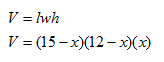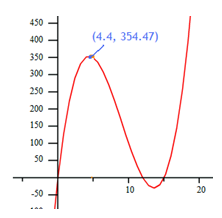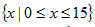Applications

There are many applications of polynomials. One of the most important is volume of a rectangular prism. Recall that the formula to determine volume of a rectangular prism is: V = lwh, where l = length, w = width and h = height.

Example Find the function that models the volume of a rectangular cereal box with length (15 - x), width (12 - x) and height (x). Determine the maximum volume of the prism.

Step 1. Use substitution to write a function in factored form.Step 2. To determine maximum volume, find the local maxima of the graph.Step 3. Determine the maximum volume for the box.

Since the local maxima is contained within the domain of this function:the maximum volume will be 354.47 cubic units.